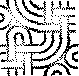#Cross Section

One of the JavaIdioms.

The memory allocated for each Java object includes a header used by the JVM.

This header includes the per-object mutex, a reference to the object's class and other information used by the heap and runtime.

When allocating a large array of ValueObjects, the memory used by the headers can be a significant overhead if each object in the array is small.

An example would be:

```	class Point2DList  {
static private final int POINT_COUNT = 10000;
private Point2D[] _points;

public Point2DList() {
_points = new Point2D[POINT_COUNT];
for( int i = 0; i < POINT_COUNT; i++ ) {
_points[i] = new Point2D.Double(0.0,0.0);
}
}

public Point2D getPoint( int n ) {
return _points[n];
}

public void setPoint( int n, Point2D p ) {
_points[n] = p;
}
}

```
The object header used by the HotSpot JVM is 8 bytes long and a double is stored in 8 bytes. Therefore the amount of memory allocated for each Point2D object is 24 bytes and the overhead for each object is 1/3 of its size!

Therefore: allocate multiple "cross section" arrays to hold what would be the instance variables of each logical object in the collection. Hide these arrays behind a FacadePattern that makes them appear to be a single array of objects that each have multiple instance variables.

For instance, the Point2DList example becomes:

```	class Point2DList {
static private final int POINT_COUNT = 10000;
private double[] _x; // The X "cross section"
private double[] _y; // The Y "cross section"

public Point2DList(){
_x = new double[POINT_COUNT];
_y = new double[POINT_COUNT];
for( int i = 0; i < POINT_COUNT; i++ ) {
_x[i] = 0.0;
_y[i] = 0.0;
}
}

public Point2D getPoint( int n ) {
return new Point2D.Double( _x[n], _y[n] );
}

public void setPoint( int n, Point2D p ) {
_x[n] = p.getX();
_y[n] = p.getY();
}
}

```
(This is a slightly contrived example because the x and y "cross sections" both have the double type and so could be stored in the same array, but you get the idea, I hope.)